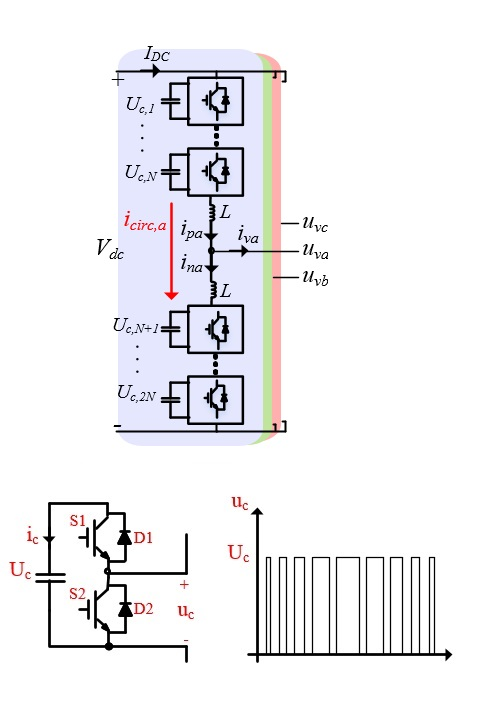LIBRARY

# Analysis of Capacitor Voltage Ripple Minimization in Modular Multilevel Converter Based on Average ModelFig. 1. Two-source and two-load-interconnected system as a benchmark for online stability monitoring.
Multilevel converters are composed of series-connected semiconductors and can operate at higher voltages than the voltage rating of the individual devices. These converters are divided into three main categories: the neutral-point clamped capacitor (NPC), the flying capacitor (FC) and the cascaded topologies, which itself is divided into cascaded H-bridge (CHB) and cascaded half-bridge or the modular multilevel converter (MMC). Multilevel converters are of special interest in applications such as active front-end rectifiers (AFE), high-voltage direct-current transmission (HVDC), static compensators (STATCOM), high-power motor drive applications, battery energy storage systems, and photovoltaic applications. The modular multilevel converter is the state-of-the-art converter among multilevel topologies and has received a huge amount of attention from researchers over past years.

In this paper, the particle swarm optimization (PSO) algorithm is used to calculate the optimal magnitude and phase angle of the circulating current components in order to minimize the voltage ripple across the capacitors. The 2nd and 4th harmonic components of the circulating current are considered to minimize the voltage ripple. The approach is based on an average model derived for the converter. For different magnitudes of arm inductance (representing different applications such as motor drives and AFEs.) the capacitor voltage waveforms are compared with the natural circulating current, eliminating the circulating current and when injecting an optimal magnitude of the circulating current. Also, the effect of the circulating current on the efficiency of the converter is investigated by calculating the semiconductor losses.